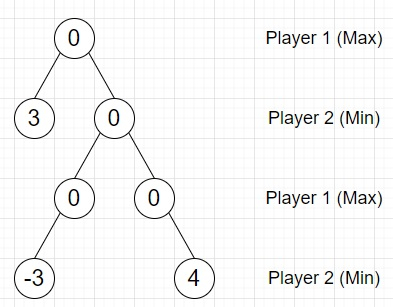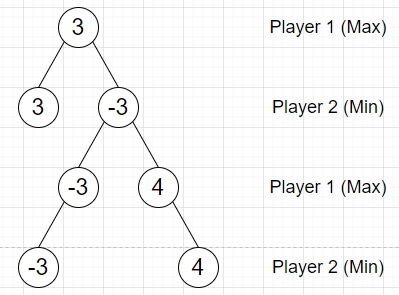# Program to fill Min-max game tree in Python

PythonServer Side ProgrammingProgramming

Suppose we have a binary tree representing a game state of a two player game. Every internal node is filled with 0 and the leaves values represent the end score. Player 1 wants to maximize the end score while player 2 wants to minimize the end score. Player 1 will always make moves on nodes at even levels and player 2 will always make moves on odd levels. We have to fill in the binary tree with the resulting scores assuming both of players play optimally.

So, if the input is likethen the output will beTo solve this, we will follow these steps −

• Define a function helper() . This will take root, h, currentHeight
• if root is empty, then
• return
• helper(left of root, h, currentHeight + 1)
• helper(right of root, h, currentHeight + 1)
• if currentHeight < h, then
• if currentHeight is even, then
• if left of root and right of root are not null, then
• value of root := maximum of value of left of root, value of right of root
• otherwise when left of root is not null, then
• value of root := value of left of root
• otherwise when right of root is not null, then
• value of root := value of right of root
• otherwise,
• if left of root and right of root are not null, then
• value of root := minimum of value of left of root, value of right of root
• otherwise when left of root is not null, then
• value of root := value of left of root
• otherwise when right of root is not null, then
• value of root := value of right of root
• Define a function height() . This will take root
• if root is null, then
• return 0
• return 1 + (maximum of height(left of root) , height(right of root))
• From the main method, do the following −
• h := height(root)
• helper(root, h, 0)
• return root

Let us see the following implementation to get better understanding −

## Example

Live Demo

class TreeNode:
def __init__(self, data, left = None, right = None):
self.val = data
self.left = left
self.right = right
class Solution:
def helper(self, root, h, currentHeight):
if not root:
return
self.helper(root.left, h, currentHeight + 1)
self.helper(root.right, h, currentHeight + 1)
if currentHeight < h:
if currentHeight % 2 == 0:
if root.left and root.right:
root.val = max(root.left.val, root.right.val)
elif root.left:
root.val = root.left.val
elif root.right:
root.val = root.right.val
else:
if root.left and root.right:
root.val = min(root.left.val, root.right.val)
elif root.left:
root.val = root.left.val
elif root.right:
root.val = root.right.val
def height(self, root):
if not root:
return 0
return 1 + max(self.height(root.left), self.height(root.right))
def solve(self, root):
h = self.height(root)
self.helper(root, h, 0)
return root
def print_tree(root):
if root is not None:
print_tree(root.left)
print(root.val, end = ', ')
print_tree(root.right)
ob = Solution()
root = TreeNode(0)
root.left = TreeNode(3)
root.right = TreeNode(0)
root.right.left = TreeNode(0)
root.right.right = TreeNode(0)
root.right.left.left = TreeNode(-3)
root.right.right.right = TreeNode(4)
print_tree(ob.solve(root))

## Input

root = TreeNode(0)
root.left = TreeNode(3)
root.right = TreeNode(0)
root.right.left = TreeNode(0)
root.right.right = TreeNode(0)
root.right.left.left = TreeNode(-3)
root.right.right.right = TreeNode(4)

## Output

3, 3, -3, -3, -3, 4, 4,